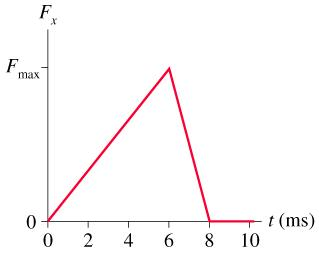# Problem: In the figure, what value of Fmax gives an impulse of 6.0 N·s?

###### FREE Expert Solution

Impulse, Jx :

$\overline{){{\mathbf{J}}}_{{\mathbf{x}}}{\mathbf{=}}{{\mathbf{F}}}_{{\mathbf{x}}}{\mathbf{t}}}$

This implies that impulse is the area under the curve.

Area of a triangle = (1/2)by###### Problem DetailsIn the figure, what value of Fmax gives an impulse of 6.0 N·s?